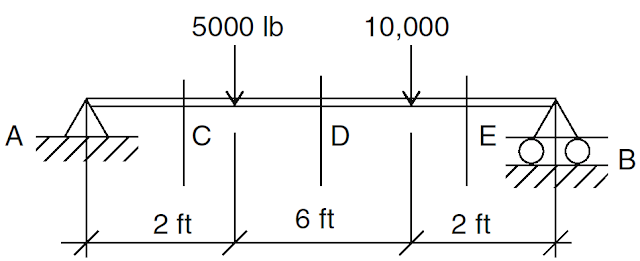# Computation of Shear Force

When a beam is subjected to applied forces, internal forces develop in the beam. Since the beam has supports and the system is in an equilibrium condition, the beam should resist these internal forces. This type of force is called shear force, or simply shear. The magnitude of the shear has a direct effect on the magnitude of the shear stress in the beam, and also on the design and analysis of the structural members.
Shear forces develop along the entire length of the beam, and their magnitude varies at each cross section of the beam. They actually act perpendicularly to the longitudinal axis of the beam. Our main objectives in finding the shear in beams are twofold. First, we have to know the maximum value of the shear in order to design the beam properly so that it can resist overall shear and will not fail when it is loaded.
The second objective is to calculate the values of shear along the length of the beam, so that we can find out where the beam fails under bending and where the shear value is zero.

As mentioned earlier, the designer must first know the magnitude of the shear force at any section of the beam to get the right picture of beam design. The magnitude of shear force at any section of the beam is equal to the sum of all the vertical forces either to the right or to the left of the section. To compute the shear force for any section of the beam, we simply call the upward forces (reactions) positive and downward forces (loads) negative. Then, the magnitude of the shear force at any section of the beam is equal to sum of the reactions minus the sum of the loads to the left of the section. This can be stated as:
Example:
Find the shear force at sections C, D, and E for the simply supported beam shown (Fig. 1).Figure 1
First, we calculate the reactions.

RA + RB = 15,000 lb

Using the moment equilibrium equation,

RA(10) - 5000(8) -10,000(2) = 0
10(RA) = 60,000
RA = 6000 lb
and
RB = 9000 lb

Point C

We usually represent a shear force by V, and we designate this shear force at C as Vc.
Vc = +6000 - 0 = 6000lb

Point D

Vd =  +6000 - 5000 = +1000 lb

Point E

Ve = +6000 - 5000 - 10,000 = -9000 lb
[blogger]

Engineeersdaily

Name

Email *

Message *